Next: Complex numbers Up: Modular mappings Previous: Real numbers

## Arbitrary powers

The signature of an arbitrary power can be computed as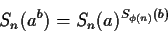where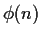is Euler's totient function or the number of divisors of n. This is necessary to guarantee that the relation Sn( ab+c ) = Sn(ab) Sn(ac). It is a consequence of Fermat's little result, that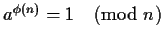. The signatures of exponents will be computed mod, a value which may have some undesirable properties. E.g.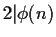for any n, and hence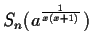cannot be computed.

For this reason it is important to find out if Sn(a) is a primitive root or not. This can be done with an efficient test. For every prime factor k of, we test whether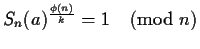. If it is 1, then Sn(a) is a kth power, and it will be a generator of at most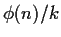values. By trying all the primes factors ofwe can find the maximum k for which. If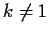signatures of the exponents should be computed (mod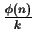). This is not only more efficient, but could remove unwanted factors fromwhich may otherwise prevent signature computation.Next: Complex numbers Up: Modular mappings Previous: Real numbers
Gaston Gonnet
1999-07-04